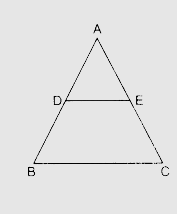# Using B.P.T., prove that a line drawn through the mid-point of one side of a triangle parallel to another side bisects the third side.

To do:

We have to prove that a line drawn through the mid-point of one side of a triangle parallel to another side bisects the third side.

Solution:

We know that,

If a line divides two sides of a triangle proportionally, then it is parallel to the third side.Let in a $\triangle ABC$, $D$ be the mid-point of $AB$ and $DE \| BC$

In $\triangle ABC, DE \| BC$,

This implies,

$\frac{AD}{DB}=\frac{AE}{EC}$.........(i)

$AD=DB$

This implies,

$\frac{AD}{AD}=\frac{AE}{EC}$

$1=\frac{AE}{EC}$

$AE = EC$

Therefore, $DE$ bisects $AC$

Hence proved.